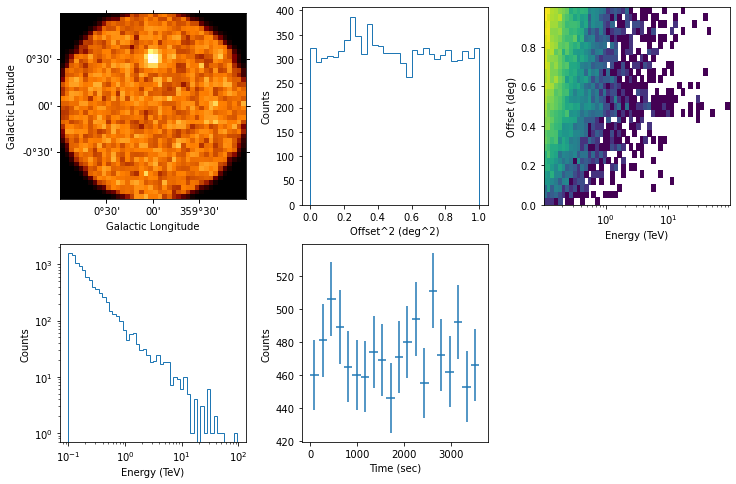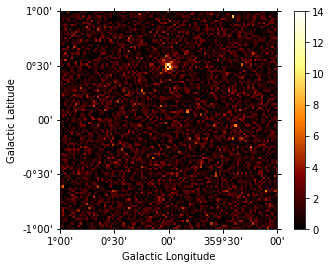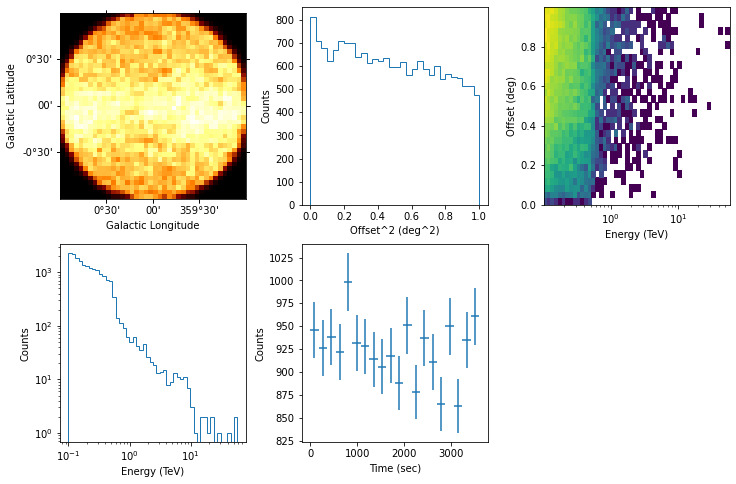This is a fixed-text formatted version of a Jupyter notebook

# Event Sampling¶

## Prerequisites¶

To understand how to generate a Model and a MapDataset, and how to fit the data, please refer to the gammapy.modeling.models.SkyModel and simulate_3d.

## Context¶

This tutorial describes how to sample events from an observation of a one (or more) gamma-ray source(s). The main aim of the tutorial will be to set the minimal configuration needed to deal with the Gammapy event-sampler and how to obtain an output photon event list.

The core of the event sampling lies into the Gammapy gammapy.datasets.MapDatasetEventSampler class, which is based on the inverse cumulative distribution function (Inverse CDF).

The gammapy.datasets.MapDatasetEventSampler takes in input a gammapy.datasets.Dataset object containing the spectral, spatial and temporal properties of the source(s) of interest.

The gammapy.datasets.MapDatasetEventSampler class evaluates the map of predicted counts (npred) per bin of the given Sky model, and the npred map is then used to sample the events. In particular, the output of the event-sampler will be a set of events having information about their true coordinates, true energies and times of arrival.

To these events, IRF corrections (i.e. PSF and energy dispersion) can also further applied in order to obtain reconstructed coordinates and energies of the sampled events.

At the end of this process, you will obtain an event-list in FITS format.

## Objective¶

Describe the process of sampling events from a given Sky model and obtaining an output event-list.

## Proposed approach¶

In this section, we will show how to define a gammapy.data.Observations and to create a gammapy.datasets.Dataset object (for more info on gammapy.datasets.Dataset objects, please visit this link). These are both necessary for the event sampling. Then, we will define the Sky model from which we sample events.

In this tutorial, we propose two examples for sampling events: one chosing a point-like source and one using a template map.

## Setup¶

:

%matplotlib inline

:

import matplotlib.pyplot as plt
from pathlib import Path
import numpy as np
import copy
import astropy.units as u
from astropy.io import fits
from astropy.coordinates import SkyCoord
from gammapy.data import DataStore, GTI, Observation
from gammapy.datasets import MapDataset, MapDatasetEventSampler
from gammapy.maps import MapAxis, WcsGeom, Map
from gammapy.makers import MapDatasetMaker
from gammapy.modeling import Fit
from gammapy.modeling.models import (
Model,
Models,
SkyModel,
PowerLawSpectralModel,
PowerLawNormSpectralModel,
PointSpatialModel,
GaussianSpatialModel,
TemplateSpatialModel,
FoVBackgroundModel,
)
from regions import CircleSkyRegion


### Define an Observation¶

You can firstly create a gammapy.data.Observations object that contains the pointing position, the GTIs and the IRF you want to consider.

Hereafter, we chose the IRF of the South configuration used for the CTA DC1 and we set the pointing position of the simulated field at the Galactic Center. We also fix the exposure time to 1 hr.

:

filename = (
"$GAMMAPY_DATA/cta-1dc/caldb/data/cta/1dc/bcf/South_z20_50h/irf_file.fits" ) pointing = SkyCoord(0.0, 0.0, frame="galactic", unit="deg") livetime = 1 * u.hr  Now you can create the observation: :  irfs = load_cta_irfs(filename) observation = Observation.create( obs_id=1001, pointing=pointing, livetime=livetime, irfs=irfs )  Invalid unit found in background table! Assuming (s-1 MeV-1 sr-1)  ### Define the MapDataset¶ Let’s generate the gammapy.datasets.Dataset object: we define the energy axes (true and reconstruncted), the migration axis and the geometry of the observation. This is a crucial point for the correct configuration of the event sampler. Indeed the spatial and energetic binning should be treaten carefully and… the finer the better. For this reason, we suggest to define the energy axes by setting a minimum binning of least 10-20 bins per decade for all the sources of interest. The spatial binning may instead be different from source to source and, at first order, it should be adopted a binning significantly smaller than the expected source size. For the examples that will be shown hereafter, we set the geometry of the dataset to a field of view of 2degx2deg and we bin the spatial map with pixels of 0.02 deg. :  energy_axis = MapAxis.from_energy_bounds( "0.1 TeV", "100 TeV", nbin=10, per_decade=True ) energy_axis_true = MapAxis.from_energy_bounds( "0.03 TeV", "300 TeV", nbin=20, per_decade=True, name="energy_true" ) migra_axis = MapAxis.from_bounds( 0.5, 2, nbin=150, node_type="edges", name="migra" ) geom = WcsGeom.create( skydir=pointing, width=(2, 2), binsz=0.02, frame="galactic", axes=[energy_axis], )  In the following, the dataset is created by selecting the effective area, background model, the PSF and the Edisp from the IRF. The dataset thus produced can be saved into a FITS file just using the write() function. We put it into the evt_sampling sub-folder: :  %%time empty = MapDataset.create( geom, energy_axis_true=energy_axis_true, migra_axis=migra_axis, name="my-dataset", ) maker = MapDatasetMaker(selection=["exposure", "background", "psf", "edisp"]) dataset = maker.run(empty, observation) Path("event_sampling").mkdir(exist_ok=True) dataset.write("./event_sampling/dataset.fits", overwrite=True)  CPU times: user 1.22 s, sys: 334 ms, total: 1.56 s Wall time: 1.62 s  ### Define the Sky model: a point-like source¶ Now let’s define a Sky model (see how to create it here) for a point-like source centered 0.5 deg far from the Galactic Center and with a power-law spectrum. We then save the model into a yaml file. :  spectral_model_pwl = PowerLawSpectralModel( index=2, amplitude="1e-12 TeV-1 cm-2 s-1", reference="1 TeV" ) spatial_model_point = PointSpatialModel( lon_0="0 deg", lat_0="0.5 deg", frame="galactic" ) sky_model_pntpwl = SkyModel( spectral_model=spectral_model_pwl, spatial_model=spatial_model_point, name="point-pwl", ) bkg_model = FoVBackgroundModel(dataset_name="my-dataset") models = Models([sky_model_pntpwl, bkg_model]) file_model = "./event_sampling/point-pwl.yaml" models.write(file_model, overwrite=True)  ### Sampling the source and background events¶ Now, we can finally add the gammapy.modeling.models.SkyModel we want to event-sample to the gammapy.datasets.Dataset container: :  dataset.models = models print(dataset.models)  DatasetModels Component 0: SkyModel Name : point-pwl Datasets names : None Spectral model type : PowerLawSpectralModel Spatial model type : PointSpatialModel Temporal model type : Parameters: index : 2.000 amplitude : 1.00e-12 1 / (cm2 s TeV) reference (frozen) : 1.000 TeV lon_0 : 0.000 deg lat_0 : 0.500 deg Component 1: FoVBackgroundModel Name : my-dataset-bkg Datasets names : ['my-dataset'] Spectral model type : PowerLawNormSpectralModel Parameters: norm : 1.000 tilt (frozen) : 0.000 reference (frozen) : 1.000 TeV  The next step shows how to sample the events with the gammapy.datasets.MapDatasetEventSampler class. The class requests a random number seed generator (that we set with random_state=0), the gammapy.datasets.Dataset and the gammapy.data.Observations object. From the latter, the gammapy.datasets.MapDatasetEventSampler class takes all the meta data information. :  %%time sampler = MapDatasetEventSampler(random_state=0) events = sampler.run(dataset, observation)  CPU times: user 168 ms, sys: 30 ms, total: 198 ms Wall time: 200 ms  The output of the event-sampler is an event list with coordinates, energies and time of arrivals of the source and background events. Source and background events are flagged by the MC_ID identifier (where 0 is the default identifier for the background). :  print(f"Source events: {(events.table['MC_ID'] == 1).sum()}") print(f"Background events: {(events.table['MC_ID'] == 0).sum()}")  Source events: 178 Background events: 11804  We can inspect the properties of the simulated events as follows: :  events.select_offset([0, 1] * u.deg).peek()By default, the gammapy.datasets.MapDatasetEventSampler fills the metadata keyword OBJECT in the event list using the first model of the SkyModel object. You can change it with the following commands: :  events.table.meta["OBJECT"] = dataset.models.name  Let’s write the event list and its GTI extension to a FITS file. We make use of fits library in astropy: :  primary_hdu = fits.PrimaryHDU() hdu_evt = fits.BinTableHDU(events.table) hdu_gti = fits.BinTableHDU(dataset.gti.table, name="GTI") hdu_all = fits.HDUList([primary_hdu, hdu_evt, hdu_gti]) hdu_all.writeto("./event_sampling/events_0001.fits", overwrite=True)  #### Generate a skymap¶ A skymap of the simulated events can be obtained with: :  counts = Map.create( frame="galactic", skydir=(0, 0.0), binsz=0.02, npix=(100, 100) ) counts.fill_events(events) counts.plot(add_cbar=True);#### Fit the simulated data¶ We can now check the sake of the event sampling by fitting the data (a tutorial of source fitting is here and here. We make use of the same gammapy.modeling.models.Models adopted for the simulation. Hence, we firstly read the gammapy.datasets.Dataset and the model file, and we fill the gammapy.datasets.Dataset with the sampled events. :  models_fit = Models.read("./event_sampling/point-pwl.yaml") counts = Map.from_geom(geom) counts.fill_events(events) dataset.counts = counts dataset.models = models_fit  Let’s fit the data and look at the results: :  %%time fit = Fit([dataset]) result = fit.run(optimize_opts={"print_level": 1}) print(result)  ------------------------------------------------------------------ | FCN = 7.136e+04 | Ncalls=102 (102 total) | | EDM = 2.3e-05 (Goal: 0.0002) | up = 1.0 | ------------------------------------------------------------------ | Valid Min. | Valid Param. | Above EDM | Reached call limit | ------------------------------------------------------------------ | True | True | False | False | ------------------------------------------------------------------ | Hesse failed | Has cov. | Accurate | Pos. def. | Forced | ------------------------------------------------------------------ | False | True | True | True | False | ------------------------------------------------------------------ OptimizeResult backend : minuit method : minuit success : True message : Optimization terminated successfully. nfev : 102 total stat : 71360.01 CPU times: user 6.8 s, sys: 951 ms, total: 7.75 s Wall time: 8.09 s  :  result.parameters.to_table()  :  Table length=8 namevalueunitminmaxfrozenerror str9float64str14float64float64boolfloat64 index1.9983e+00nannanFalse6.315e-02 amplitude1.0429e-12cm-2 s-1 TeV-1nannanFalse9.895e-14 reference1.0000e+00TeVnannanTrue0.000e+00 lon_01.3912e-03degnannanFalse3.396e-03 lat_05.0228e-01deg-9.000e+019.000e+01False3.295e-03 norm9.8943e-01nannanFalse9.154e-03 tilt0.0000e+00nannanTrue0.000e+00 reference1.0000e+00TeVnannanTrue0.000e+00 The results looks great! ## Extended source using a template¶ The event sampler can also work with a template model. Here we use the interstellar emission model map of the Fermi 3FHL, which can be found in the GAMMAPY data repository. We proceed following the same steps showed above and we finally have a look at the event’s properties: :  template_model = TemplateSpatialModel.read( "$GAMMAPY_DATA/fermi-3fhl-gc/gll_iem_v06_gc.fits.gz", normalize=False
)
# we make the model brighter artificially so that it becomes visible over the background
diffuse = SkyModel(
spectral_model=PowerLawNormSpectralModel(norm=5),
spatial_model=template_model,
name="template-model",
)

bkg_model = FoVBackgroundModel(dataset_name="my-dataset")

models_diffuse = Models([diffuse, bkg_model])

file_model = "./event_sampling/diffuse.yaml"
models_diffuse.write(file_model, overwrite=True)

WARNING: FITSFixedWarning: 'datfix' made the change 'Set DATE-REF to '1858-11-17' from MJD-REF'. [astropy.wcs.wcs]

:

dataset.models = models_diffuse
print(dataset.models)

DatasetModels

Component 0: SkyModel

Name                      : template-model
Datasets names            : None
Spectral model type       : PowerLawNormSpectralModel
Spatial  model type       : TemplateSpatialModel
Temporal model type       :
Parameters:
norm                    :   5.000
tilt         (frozen)   :   0.000
reference    (frozen)   :   1.000  TeV

Component 1: FoVBackgroundModel

Name                      : my-dataset-bkg
Datasets names            : ['my-dataset']
Spectral model type       : PowerLawNormSpectralModel
Parameters:
norm                    :   1.000
tilt         (frozen)   :   0.000
reference    (frozen)   :   1.000  TeV


:

%%time
sampler = MapDatasetEventSampler(random_state=0)
events = sampler.run(dataset, observation)

CPU times: user 3.59 s, sys: 788 ms, total: 4.38 s
Wall time: 4.41 s

:

events.select_offset([0, 1] * u.deg).peek()### Simulate mutiple event list¶

In some user case, you may want to sample events from a number of observations. In this section, we show how to simulate a set of event lists. For simplicity we consider only one point-like source, observed three times for 1 hr and assuming the same pointing position.

Let’s firstly define the time start and the livetime of each observation:

:

tstarts = [1, 5, 7] * u.hr
livetimes = [1, 1, 1] * u.hr

:

%%time
for idx, tstart in enumerate(tstarts):

observation = Observation.create(
obs_id=idx,
pointing=pointing,
tstart=tstart,
livetime=livetimes[idx],
irfs=irfs,
)

dataset = maker.run(empty, observation)
dataset.models = models

sampler = MapDatasetEventSampler(random_state=idx)
events = sampler.run(dataset, observation)
events.table.write(
f"./event_sampling/events_{idx:04d}.fits", overwrite=True
)

CPU times: user 6.06 s, sys: 1.3 s, total: 7.36 s
Wall time: 7.03 s


You can now load the event list with Datastore.from_events_files() and make your own analysis following the instructions in the analysis_2 <analysis_2.ipynb>__ tutorial.

:

path = Path("./event_sampling/")
paths = list(path.rglob("events*.fits"))
data_store = DataStore.from_events_files(paths)
data_store.obs_table

:

ObservationTable length=3